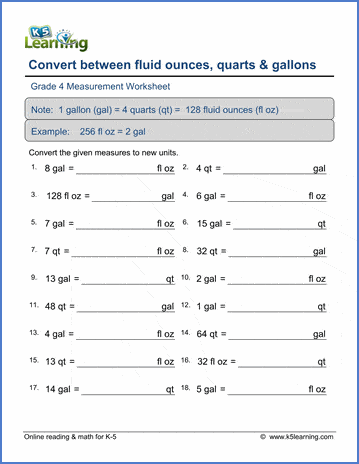# 4th Grade Math Worksheets: Measurement

## Measurement worksheets

These worksheets review the customary and metric units for measuring length, weight, capacity and temperature. The object is to improve familiarity with the different units and provide practice converting measurements between different units of the same system (e.g. inches to feet). Conversion between systems is not covered until grade 5.

## Length

Units of length (inches, feet, yards & miles)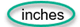Metric units of length (mm, cm, m & km)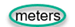Reading rulers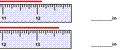Comparing lengths 60 in  >  4 ft

Convert inches, feet and yards 9 ft 6 in = ______ in
Convert miles into feet or yards 2 mi = _____ ft

Convert mm, cm, m, and km 8 km 713 m= ___ m
Convert mm, cm, m, and km (decimals) 5.5 cm = ____ mm

## Weight

Units of weight (ounces, pounds and tons)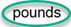Metric units of weight (g & kg)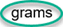Reading scales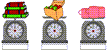Balancing a scale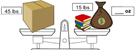Comparing weights 3 lbs > 45 oz

Convert ounces, pounds, and tons 1 lb 2 oz = _____ oz

Convert g & kg 3.146 kg = ______ g

## Capacity

Units of capacity (teaspoons to gallons)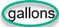Metric units of capacity (ml & L)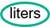Capacity charts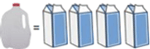Reading measuring cups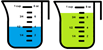Comparing capacities 6 gal < 25 qt

Convert between cups, pints, quarts and gallons 32 qt = ____ gal
Convert between ounces, cups and quarts 24 oz = _____ c
Convert between ounces, quarts and gallons 3 gal = ____ oz

Capacities with fractional amounts 4 1/2 qt = 9 pints
Convert between ml & l  4.3 L=  ____ ml

## Temperature

Understanding Fahrenheit and Celsius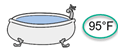Converting between Fahrenheit and Celsius 12 °C = _____° F

## Mixed units

Units of measurement in, ft, yd or m?
Estimating measurements 4 m or 4 km?

Mixed conversions (customary units) 65 oz = ____ gal
Mixed conversions (metric units) 6.7 m = ____ cm

Metric abbreviations Word problems
Measurement projects Project

## Measurement word problems

Length word problems Word problems
Mass and weight word problems Word problems
Volume and capacity word problems Word problems
Time word problems Word problems
Money word problems Word problems